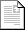ASEE Zone 2 Conference 2017

Proceedings »

Methods to Improve Students Learning in Dynamic Systems and Control Course

 Final Paperpdf238KB

Study of Dynamic Systems involve modeling and analysis of system response to different inputs. Mathematical models of electrical and mechanical systems consist of first order and second order differential equations. To find the response of such systems, students need to solve the resulting modeling equations. Conventional methods using trial solution approach may sometimes be frustrating for the students if the model is complex. Laplace transform method do help by converting the differential equations into algebraic equations but that method is also not very helpful when it comes to solving multi degree of freedom systems. Using MATLAB simulations to solve these modeling equations make life easier for students and is a subject of this paper. While MATLAB do not replace the need for theoretical teaching, it offers a quick solution to increase students’ engagement and comprehension. This method of teaching is adapted for the first time this semester and needs further investigation of its popularity and its impact on students’ result but midterm evaluation feedback from students suggest that these simulations are providing an effective and enjoyable source of learning.

Author(s):

Arjumand Ali
School of Engineering
Grand Valley State University
United States